# Quiz 28: Managing Aggregate Demand: Fiscal Policy

In Table (2), the GDP of an economy equals to consumption expenditure when both are at \$1,720; hence, the equilibrium level of GDP is \$1,720. Graphical presentation of equilibrium level of GDP In Figure (1), GDP is measured on horizontal axis and consumption expenditure is measured on vertical axis.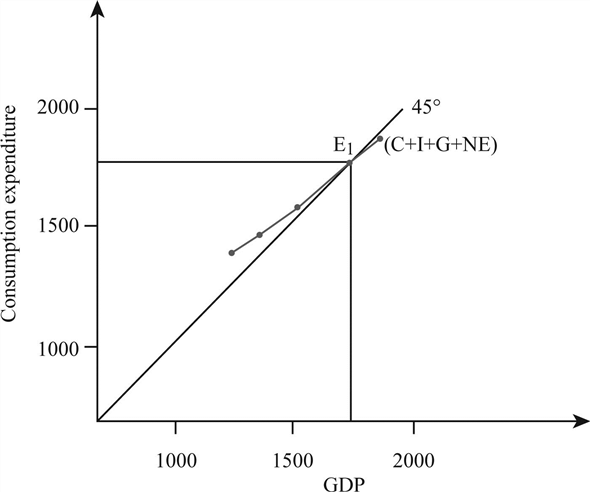Figure - 1 The above figure explains that the consumption expenditure and GDP obtain equilibrium at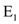when both values are at \$1,720 and intersects the 45-degree line. The marginal propensity to consume (MPC) explains the increase in personal consumption per increase in additional amount of disposable income. The marginal propensity to consume can be calculated using the following formula: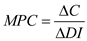……(1) The following table presents marginal propensity to consume using the formula in Equation (1). Table - 3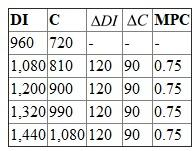Fifth column of Table (1) shows the marginal propensity to consume is \$0.75. The consumption multiplier can be calculated using the following formula: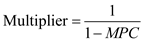……(2) Using Equation (2), calculate the multiplier:The result reveals that if 75 percent of the income is consumed, then its multiplier effect on income will be 4 percent. Since the multiplier effect of consumption is 4, the reduction in government purchases by \$60 will reduce the consumption expenditure by \$240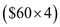.